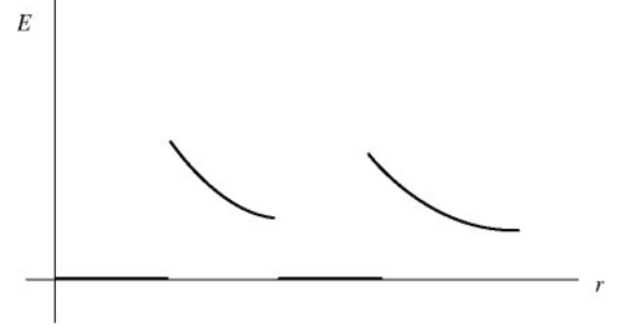# Problem: The sketch below represents a graph that shows the electric field strength (not the field lines) as a function of distance from the center for a pair of concentric uniformly charged spheres. Which of the following situations could the graph plausibly represent? A. a solid nonconducting sphere, uniformly charged throughout its volume, inside of a positively charged conducting sphereB. a positively charged nonconducting thin-walled spherical shell inside of a negatively charged conducting sphere C. a positively charged conducting sphere within an uncharged conducting sphere D. a positively charged conducting sphere within another positively charged conducting sphereE. a positively charged nonconducting thin-walled spherical shell inside of another positively charged nonconducting thin-walled spherical shell

###### FREE Expert Solution

The graph has regions where the electric field is zero.

Using Gauss law:

E•A = Qenc0

E = Qenc0A. This expression implies that the electric field will be zero if the net charge enclosed is zero.

Besides, the electric field inside a conducting material is zero.

93% (490 ratings)###### Problem Details

The sketch below represents a graph that shows the electric field strength (not the field lines) as a function of distance from the center for a pair of concentric uniformly charged spheres. Which of the following situations could the graph plausibly represent?A. a solid nonconducting sphere, uniformly charged throughout its volume, inside of a positively charged conducting sphere

B. a positively charged nonconducting thin-walled spherical shell inside of a negatively charged conducting sphere

C. a positively charged conducting sphere within an uncharged conducting sphere

D. a positively charged conducting sphere within another positively charged conducting sphere

E. a positively charged nonconducting thin-walled spherical shell inside of another positively charged nonconducting thin-walled spherical shell

Frequently Asked Questions

What scientific concept do you need to know in order to solve this problem?

Our tutors have indicated that to solve this problem you will need to apply the Electric Fields with Calculus concept. You can view video lessons to learn Electric Fields with Calculus. Or if you need more Electric Fields with Calculus practice, you can also practice Electric Fields with Calculus practice problems.